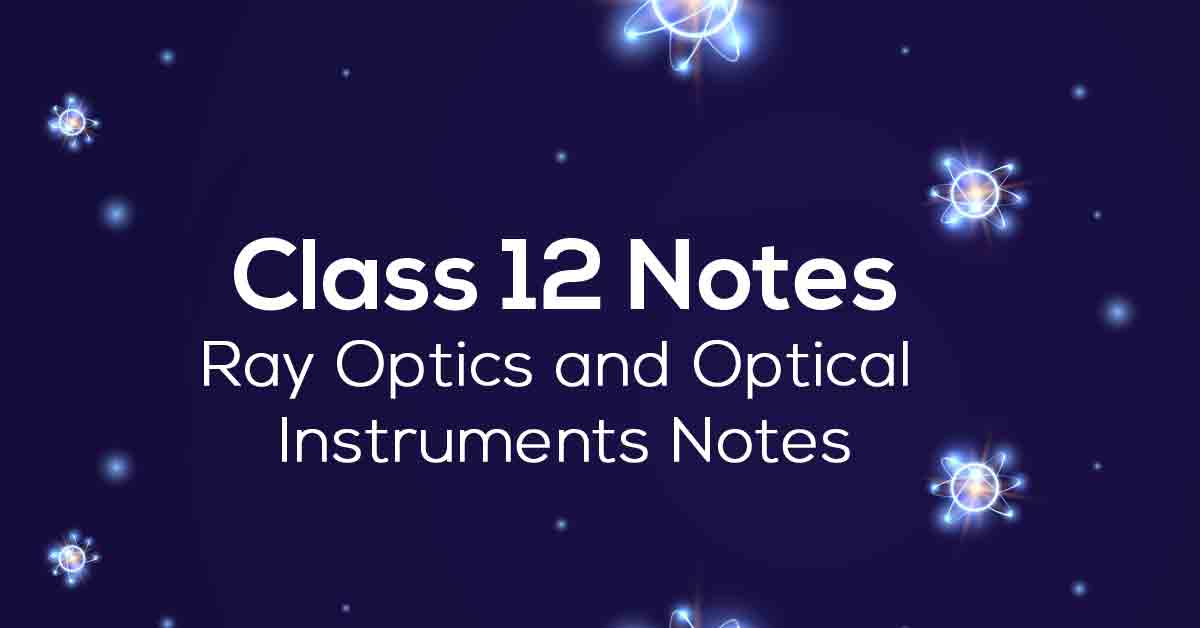Class 12th Physics Ray Optics and Optical Instruments NCERT Notes CBSE 2023

# Ray Optics and Optical Instruments Class 12 Notes# Chapter 9 Ray Optics and Optical Instruments

NCERT notes for Physics Grade 12 Chapter 9 provided by Vidyakul is the best study material for the exam. Chapter Light ray optics is based on the properties of light as it passes through convex and concave lenses. In addition, this chapter uses ray diagrams to demonstrate the linear propagation of light.

Internal total reflection and lens power are some of the important topics covered in detail in this chapter. In addition, the Vidyakul Explainer benefits students in preparing for the exam as they explore new topics.

### Points to Remember

Some of the important points to remember from this chapter are as follows:

• The phenomenon of changing the path of light without changing the medium is known as reflection.

• Therefore, a reflection of light is the return of light in the same medium from which it came after striking a surface.

• Total internal reflection occurs when light is completely reflected as it travels from a denser medium to a rarer medium.

• The focal length of the eye lens can be adjusted so that the image is always formed on the retina by the eye’s ability known as accommodation.

• Also, the incident, reflected, and normal rays exist in the same plane.

• Moreover, distances measured parallel to the incident light are positive; while distances measured perpendicular to the incident light are negative.

• The focal length (f) of a concave mirror is negative, while it is positive for a convex mirror.

• The splitting of light into its constituent colors is known as dispersion.

• Also, the medium’s refractive index is affected by the frequency of the light.

### Topics and Sub-topics

NCERT Grade 12 Physics Chapter 9 are very important notes to prepare for the CBSE board exam. This chapter helps to understand images formed by spherical lenses and spherical mirrors. In addition to these topics, it also discusses the focal length of a spherical mirror.

The three phenomena of light namely reflection, refraction, and dispersion are explained in detail in this chapter. Finally, the chapter deals with the eye, the microscope, and the telescope. So step up your exam preparation with Vidyakul's AI assistance.

Below we have provided the important topics covered in CBSE Class 12 Physics Chapter 9:

 Topic Name Topic Name Reflection of Light by Spherical Mirrors Combination of Thin Lenses in Contact Sign Convention Refraction Through a Prism Focal Length of Spherical Mirrors Dispersion by a Prism The Mirror Equation Some Natural Phenomena Due to Sunlight Refraction The Rainbow Total Internal Reflection Scattering of Light Refraction at Spherical Surfaces and by Lenses Optical Instruments Refraction at a Spherical Surface The Eye Refraction by a Lens The Microscope Power of a Lens Telescope

Download Vidyakul App for more Important videos, PDF's and Free video lectures.

### Few Important Questions

• What is the ‘Len’s formula’?

According to the Convex Lens equation, the Lens formula is 1/f = 1/v + 1/u. It relates the Focal Length of a Lens with the distance of an object which is placed in front of it and the image formed of that object.

• What are the uses of ‘Telescope’?

There are three basic functions of a telescope: light gathering, resolving, and magnifying.

• What is ‘Ray Optics’?

Geometrical optics, or ray optics, is a model of optics that describes light propagation in terms of rays.

### Practice Questions

1. The size of a small candle is 4.5 cm and is placed 30 cm in front of a concave mirror with a radius of curvature of 40 cm. In order to obtain a sharp image, the distance from the mirror where the screen should be placed is? If the candle is moved closer to the mirror, how would the screen have to be moved Describe the nature and size of the image?

2. In front of a convex mirror of focal length 20 cm, a needle of 5 cm is placed at a distance of 15 cm from it. Find out what happens as the needle is moved farther from the mirror. Find out the location of the image and its magnification.

3. What is the refractive index of water when a tank is filled with water to a height of 15 cm? The apparent depth of a needle lying at the bottom of the tank is 10 cm. If water is replaced by a liquid of refractive index 2 up to the same height, by what distance would the microscope have to be moved to focus on the needle again?